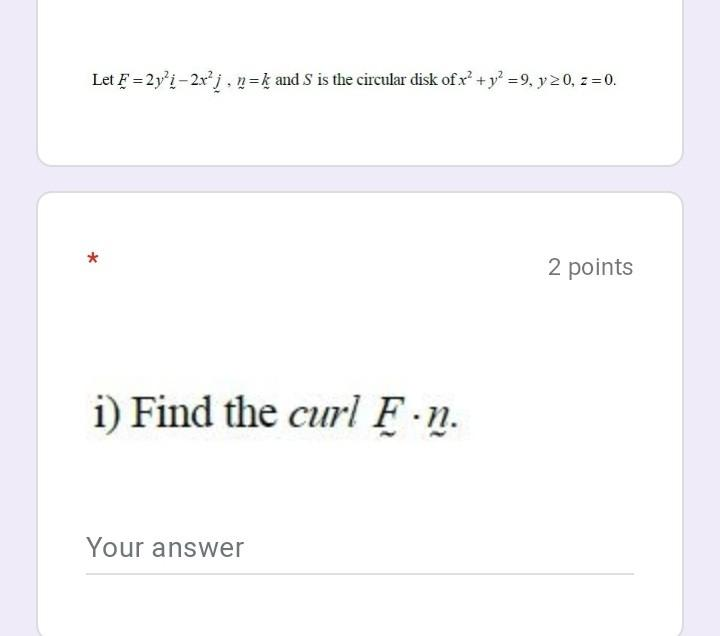### Create an Account

Already have account?

### Forgot Your Password ?

Home / Questions / Let F =2y’i – 2x’j, n=k and S is the circular disk of x' + y2 =9, y 20, z = 0. 2 points i)...

# Let F =2y’i – 2x’j, n=k and S is the circular disk of x' + y2 =9, y 20, z = 0. 2 points i) Find the curl F.n. Your answer

Let F =2y’i – 2x’j, n=k and S is the circular disk of x' + y2 =9, y 20, z = 0. 2 points i) Find the curl F.n. Your answerApr 14 2021 View more View Less

#### Answer (Solved)Subscribe To Get Solution